Function Repository Resource:

SymbolToPolygon

Return a polygon, given a symbol

Contributed by: Ed Pegg Jr
 ResourceFunction["SymbolToPolygon"][symbol,dimension] returns symbol as a polygon with vertices in dimension.

Details

The value of dimension can be "2D" or "3D" (the default).
The first argument can also be a String.

Examples

Basic Examples (2)

Label the sides of a cube:

 In:=Out=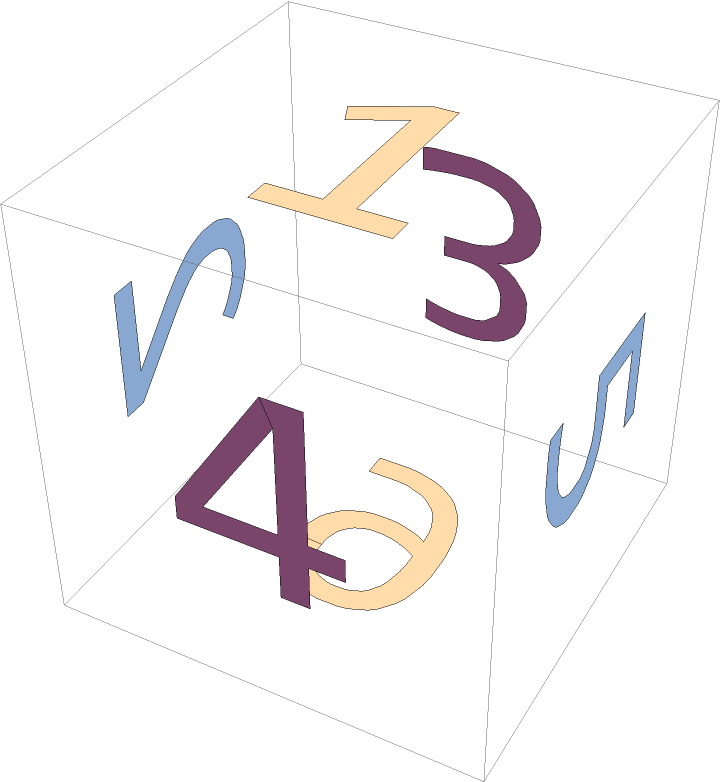Return a 2D polygon for 42:

 In:=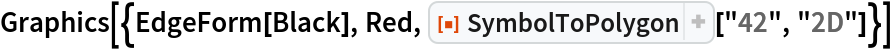Out=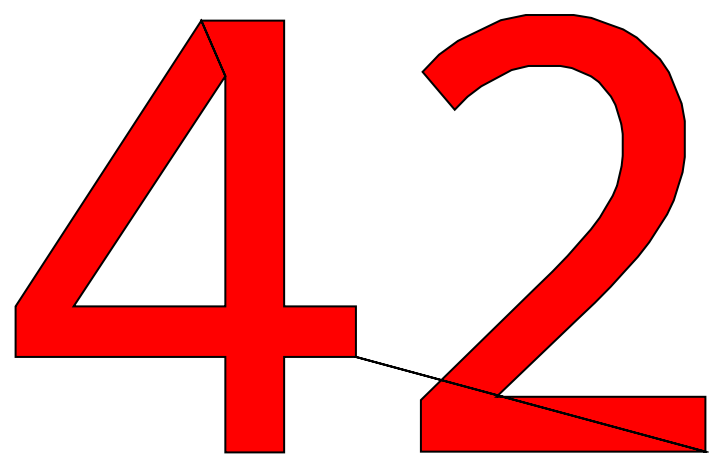Scope (1)

Different styles and fonts can be used:

 In:=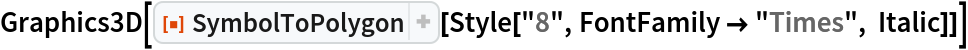Out=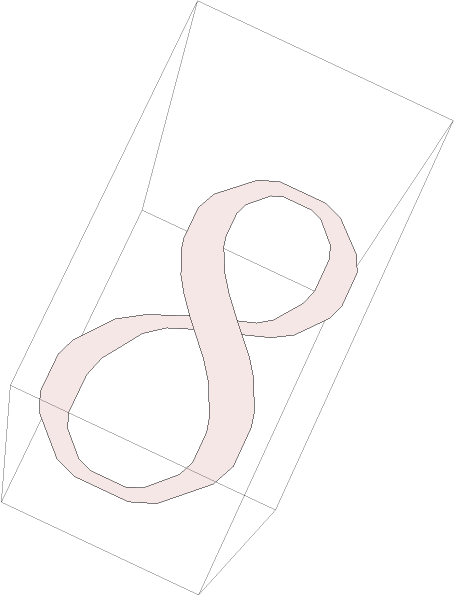Requirements

Wolfram Language 11.3 (March 2018) or above